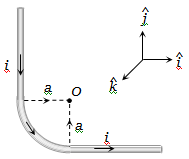NEET Physics Moving Charges and Magnetism Questions Solved

The unit vectors  are as shown below. What will be the magnetic field at O in the following figure(a) $\frac{{\mu }_{0}}{4\mathrm{\pi }}\frac{i}{a}\left(2-\frac{\mathrm{\pi }}{2}\right)\stackrel{^}{j}$                               (b) $\frac{{\mu }_{0}}{4\mathrm{\pi }}\frac{i}{a}\left(2+\frac{\mathrm{\pi }}{2}\right)\stackrel{^}{j}$

(c) $\frac{{\mu }_{0}}{4\mathrm{\pi }}\frac{i}{a}\left(2+\frac{\mathrm{\pi }}{2}\right)\stackrel{^}{i}$                                (d) $\frac{{\mu }_{0}}{4\mathrm{\pi }}\frac{i}{a}\left(2+\frac{\mathrm{\pi }}{2}\right)\stackrel{^}{k}$

Explanation is a part of a Paid Course. To view Explanation Please buy the course.

Difficulty Level: